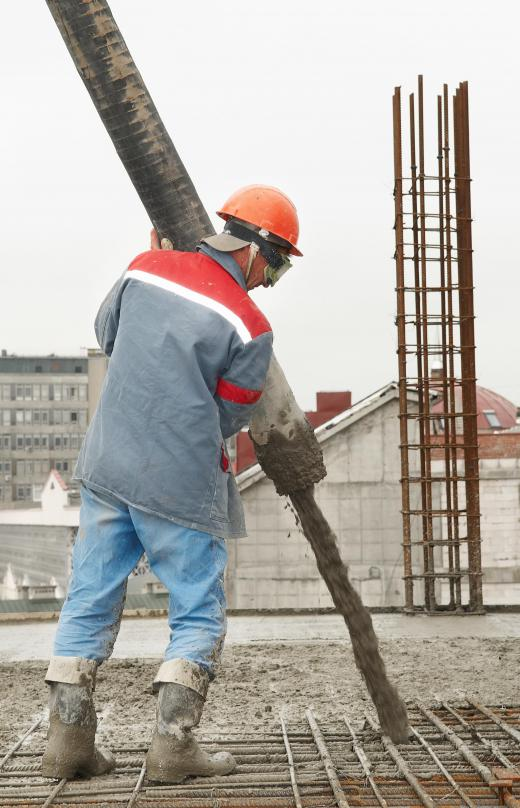Science
Fact Checked

# What is Modulus of Elasticity?

Dorothy Distefano
Dorothy Distefano

Modulus of elasticity, also known as elastic modulus or Young’s Modulus, is a measure of how a material or structure will deform and strain when placed under stress. Materials deform differently when loads and stresses are applied, and the relationship between stress and strain typically varies. The ability of matter to resist or transmit stress is important, and this property is often used to determine if a particular material is suitable for a specific purpose.

This property is often determined in a laboratory, using an experimental technique known as tensile testing, which is usually conducted on a sample of material with a specific shape and dimensions. There are a variety of testing devices available that apply very precise loads and stresses to the sample, and accurately measure and record any resulting strain in the material. The modulus of elasticity is known for a wide variety of structural materials, including metals, wood, glass, rubber, ceramics, concrete, and plastics.Engineers need to understand the strength and elasticity of structures and materials.

The modulus of elasticity describes the relationship between the stress applied to a material and its corresponding strain. Stress is defined as a force applied over a unit area, with typical units of pounds per square inch (psi) or Newtons per square meter — also known as pascals (Pa). Strain is a measure of the amount that a material deforms when stress is applied and is calculated by measuring the amount of deformation when under stress, as compared to the matter's original dimensions. Modulus of elasticity is based on Hooke’s Law of elasticity and can be calculated by dividing the stress by the strain.A type measurement, modulus of elasticity can be used to determine how much stress cured concrete can take before degrading or deforming.

For many materials at low levels of stress and under tension, the stress and strain are proportional — meaning they increase and decrease in a constant way, relative to each other. Deformation of a material that occurs when the stress and strain behave proportionally is known as elastic deformation or elastic strain. Modulus of elasticity describes the relationship between stress and strain when under these conditions.

Elasticity is the ability of a material to return to its original state or dimensions after a load, or stress, is removed. Elastic strain is reversible, meaning the strain will disappear after the stress is removed and the material will return to its original state. Materials that are exposed to intense levels of stress may deform to the point where the stress and strain no longer behave proportionally, and the material will not return to its original dimensions. This is referred to as plastic deformation or plastic strain.

## You might also Likeanon938139

@matthewc23: The machine that we most commonly use in my lab is the Instron 600 kn Hydraulic Testing System. It applies and relieves a load within a specific range (2 percent to 10 percent of the minimum breaking load when using the ISO 9856 standard test method). The test must run for a minimum of 200 cycles in this range, and from this you can determine your modulus, your elastic elongation, as well as your permanent elongation for the material being tested. A variety of different sample sizes can be tested, and are variables in the final calculations. The thickness of the sample is also important.

matthewc23

What is the strongest material on earth? I would assume it is some type of synthetic material, but I could be wrong.

When researchers do modulus of elasticity tests, what kind of machine do they use? I think it would be very interesting to see how the different tests are done and how they are measured.

I also wonder if there is a special term for the point when a material like glass starts to split into shards when you pull it apart. I assume everything would do this to some extent, but glass would be the most obvious I think.

TreeMan

@Izzy78 and @Emilski - When I was in college, I actually worked a laboratory that did a lot of these tests. This is the first time I've revisited the modulus of elasticity for quite some time, but I think I still remember a lot of it.

The answer the first question, there is a special organization that sets standards for nearly every type of laboratory test that affects building materials. That way every scientist is on the same page.

The lab I worked in mostly tested wood, but we did some tests with other materials for comparisons. I don't remember the exact psi measures, but metals were always stronger than wood with steel being the strongest we tested.

As for other tests, we did several tests that looked at compression, and bending resistance. Some of the more interesting tests involved seeing how much pressure railroad ties could withstand.

Izzy78

@Emilski - I would be interested to know a lot of the same things. I'm sure even between different materials there would be a huge amount of difference. Just think about all the types of wood there are or all of the types of metal.

Besides the modulus of elasticity, what are some of the other measures that are taken to determine the strength of an object?

Emilski

Are there different protocols for how various materials are tested? If you were working in a lab, wouldn't you need to make sure you were doing the same tests as everyone else so that you could compare results?

How do different materials compare with each other? I'm really curious about the differences between different materials and their ability to respond to bending compared to pulling or pushing. Does anyone know as a general rule how various materials rank?